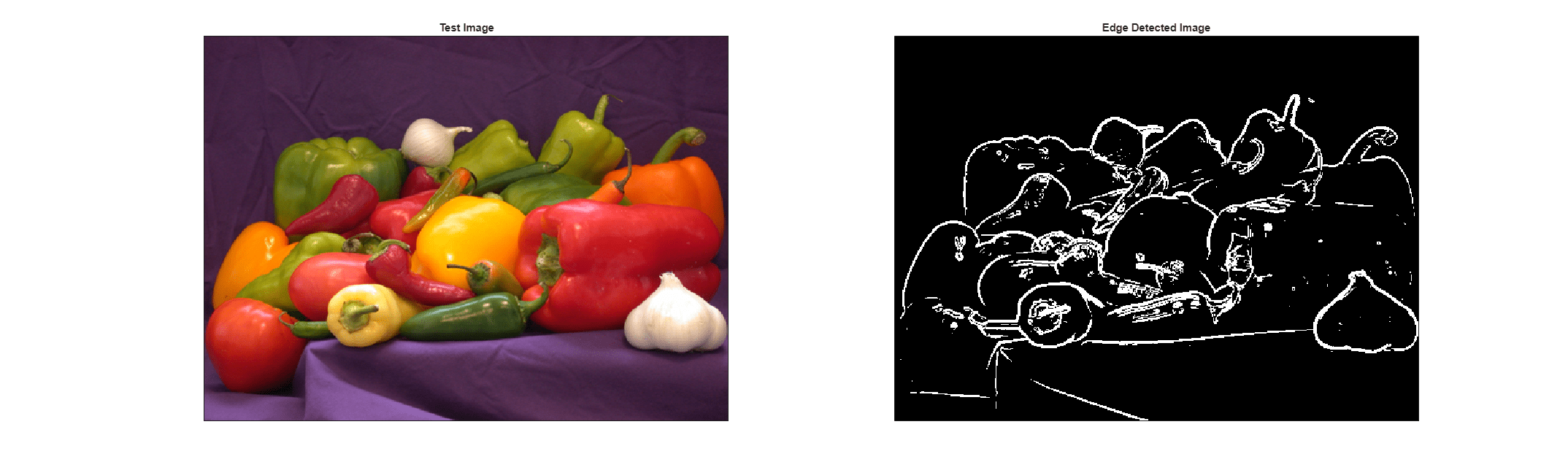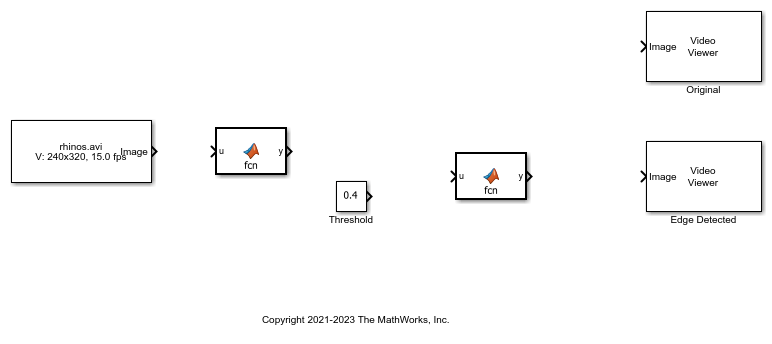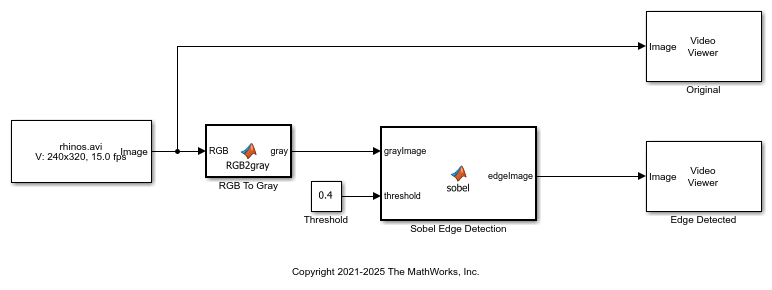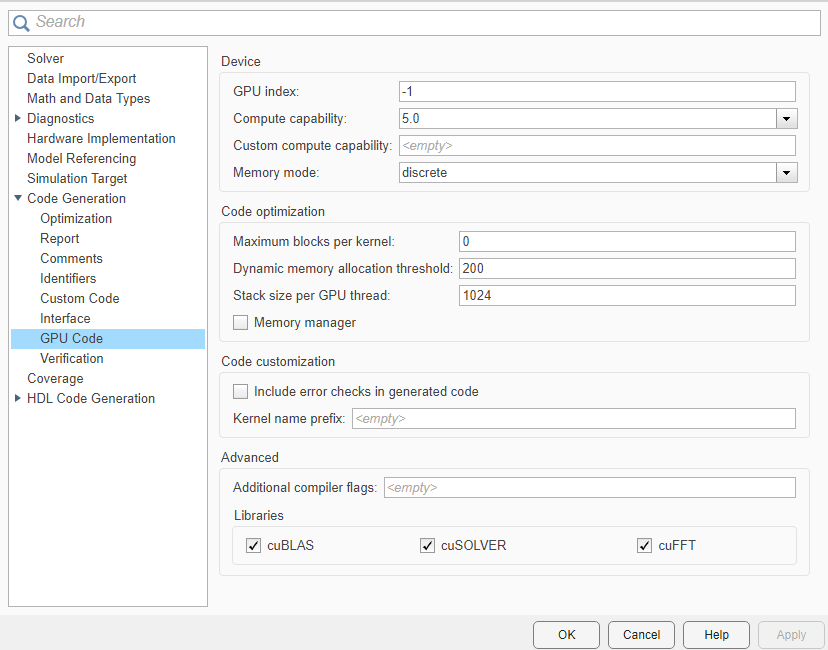## Code Generation from Simulink Models with GPU Coder

GPU Coder™ generates optimized CUDA® code from Simulink® models containing MATLAB Function blocks. You can use the generated code and executable for rapid prototyping on NVIDIA® GPUs. Code generation reports and traceability enable you to view and analyze the generated code.

To generate CUDA code, you:

• Create or open a model. Move the computationally intensive portions of your application into MATLAB Function blocks

• Select the Solver, Language, and other GPU-specific configuration parameters.

• Build the model.

### Generate CUDA Code For Sobel Edge Detection

This example shows how to generate CUDA® code for a Sobel edge detection model. The edge detection model uses MATLAB Function blocks to implement the detection algorithm.

Sobel Edge Detection

The Sobel edge detection algorithm performs a 2-D spatial gradient operation on a grayscale image. This algorithm emphasizes the high spatial frequency regions that correspond to the edges of the input image.

The Sobel edge algorithm computes the horizontal gradient, `H` and the vertical gradient, `V` of the input image by using two orthogonal filter kernels, `k` and `k'`. After the filtering operation, the algorithm computes the gradient magnitude and applies a threshold to find the regions of the images identified as edges.

```img = imread('peppers.png'); threshold = 100; k = single([1 2 1; 0 0 0; -1 -2 -1]); H = conv2(img(:,:,2),k, 'same'); V = conv2(img(:,:,2),k','same'); E = sqrt(H.*H + V.*V); edgeImage = uint8((E > threshold) * 255); h = figure; h.Position(3) = 2*h.Position(3); ax1 = subplot(1,2,1); ax2 = subplot(1,2,2); image(ax1,img); xticks(ax1,[]); yticks(ax1,[]) title(ax1,'Test Image') image(ax2,repmat(edgeImage,[1 1 3])); xticks(ax2,[]); yticks(ax2,[]) title(ax2,'Edge Detected Image') ```Create Edge Detection Model

1. Create a Simulink model and insert two MATLAB Function blocks from the User-Defined Functions library.

2. Add a Constant block and set its value to `0.4`.

3. Add a From Multimedia File block from the Computer Vision Toolbox™ library.

4. Open the From Multimedia File block and set the File name parameter to `rhinos.avi`. Set the Image signal parameter to `One multidimensional signal`.

5. Add two Video Viewer blocks from the Computer Vision Toolbox library to the model.

To open a model that contains these blocks, enter:

```open_system("edgeDetectionInitial") ```6. Double-click one of the MATLAB Function blocks. A default function signature appears in the MATLAB Function Block Editor.

7. Define a function called `sobel` that implements the Sobel edge detection algorithm. The function header declares `grayImage` and `threshold` as input arguments to the `sobel` function and `edgeImage` as the return value.

Save Editor document.

```function edgeImage = sobel(grayImage,threshold) %#codegen ```
```% Define Kernel for Sobel edge detection k = single([1 2 1; 0 0 0; -1 -2 -1]); ```
```% Detect Edge H = conv2(single(grayImage),k, 'same'); V = conv2(single(grayImage),k','same'); E = sqrt(H.*H + V.*V); ```
```edgeImage = uint8((E > threshold) * 255); ```
```end ```

8. Open the block parameters for the MATLAB Function block. On the Code Generation tab, set the Function packaging parameter to `Reusable function`. If the Function packaging parameter is set to any other value, CUDA kernels may not get generated.

9. Modify the other MATLAB Function block to implement the RGB-to-grayscale conversion. Set the Function packaging parameter of the MATLAB Function block to `Reusable function`.

```function gray = RGB2gray(RGB) %#codegen ```
```% Convert color image to grey image gray = (0.2989 * double(RGB(:,:,1)) + ... 0.5870 * double(RGB(:,:,2)) + ... 0.1140 * double(RGB(:,:,3))); ```
```end ```

10. Connect these blocks as shown in the diagram. Save the model as `edgeDetection.slx`. To open a preconfigured model, enter:

```open_system("edgeDetection"); ```11. To test the model for errors, simulate the model. On the toolstrip, click Run.

To see all video frames during simulation, open the Video Viewer block and disable Simulation > Drop Frames to Improve Performance.

```set_param('edgeDetection', 'SimulationMode', 'Normal'); sim('edgeDetection'); ```Configure Model for Code Generation

The model configuration parameters provides options for the code generation and build process.

1. Open the Configuration Parameters dialog box. Open the Solver pane. To compile your model for acceleration and generate CUDA code, configure the model to use a fixed-step solver. This table shows the solver configuration for this example.

2. In the left-pane, click Code Generation, then set the System target file to `grt.tlc`.

You can also use the Embedded Coder® target file `ert.tlc` or a custom system target file.

For GPU code generation, the custom target file must be based on `grt.tlc` or `ert.tlc`. For information on developing a custom target file, see Customize System Target Files (Simulink Coder).

3. Set the Language to `C++`.

4. Select Generate GPU code.

5. Select Generate code only.

6. Select the Toolchain. For Linux® platforms, select `NVIDIA CUDA | gmake (64-bit Linux)`. For Windows® systems, select `NVIDIA CUDA (w/Microsoft Visual C++ 20XX) | nmake (64-bit windows)`.

When using a custom system target file, you must set the build controls for the toolchain approach. To learn more about toolchain approach for custom targets, see Support Toolchain Approach with Custom Target (Simulink Coder).

7. Click Code Generation > Interface and disable MAT-file logging.

8. Click Code Generation > Report and select Create code generation report and Open report automatically.

9. Optionally, open Code Generation > GPU Code to view and edit GPU-specific options. For this example, you can use the default values of these parameters.10. Click OK to save and close the Configuration Parameters dialog box.

Alternatively, use the `set_param` function to configure the model parameters programmatically. For example,to set the Generate GPU Code parameter, enter:

```set_param('edgeDetection','GenerateGPUCode','CUDA'); ```

Generate CUDA Code for the Model

1. Open the Simulink Coder app and click Generate code.

Messages appear in the Diagnostics Viewer. The code generator produces CUDA source and header files, and an HTML code generation report. The code generator places the files in a build folder, a subfolder named `edgeDetection_grt_rtw` under your current working folder.

You can find the CUDA kernels in the `model_name_eML_blk_kernel` and `model_name_eML_blk_kernel_c` functions. The information within the triple chevrons is the execution configuration for the kernel.

### Limitations

• GPU code generation for MATLAB Function in Stateflow® charts is not supported.

• The MATLAB Function block does not support all the data types from the MATLAB® language. For supported data types, see MATLAB Function (Simulink).

• For GPU code generation, the custom target file must be based on `grt.tlc` or `ert.tlc`.